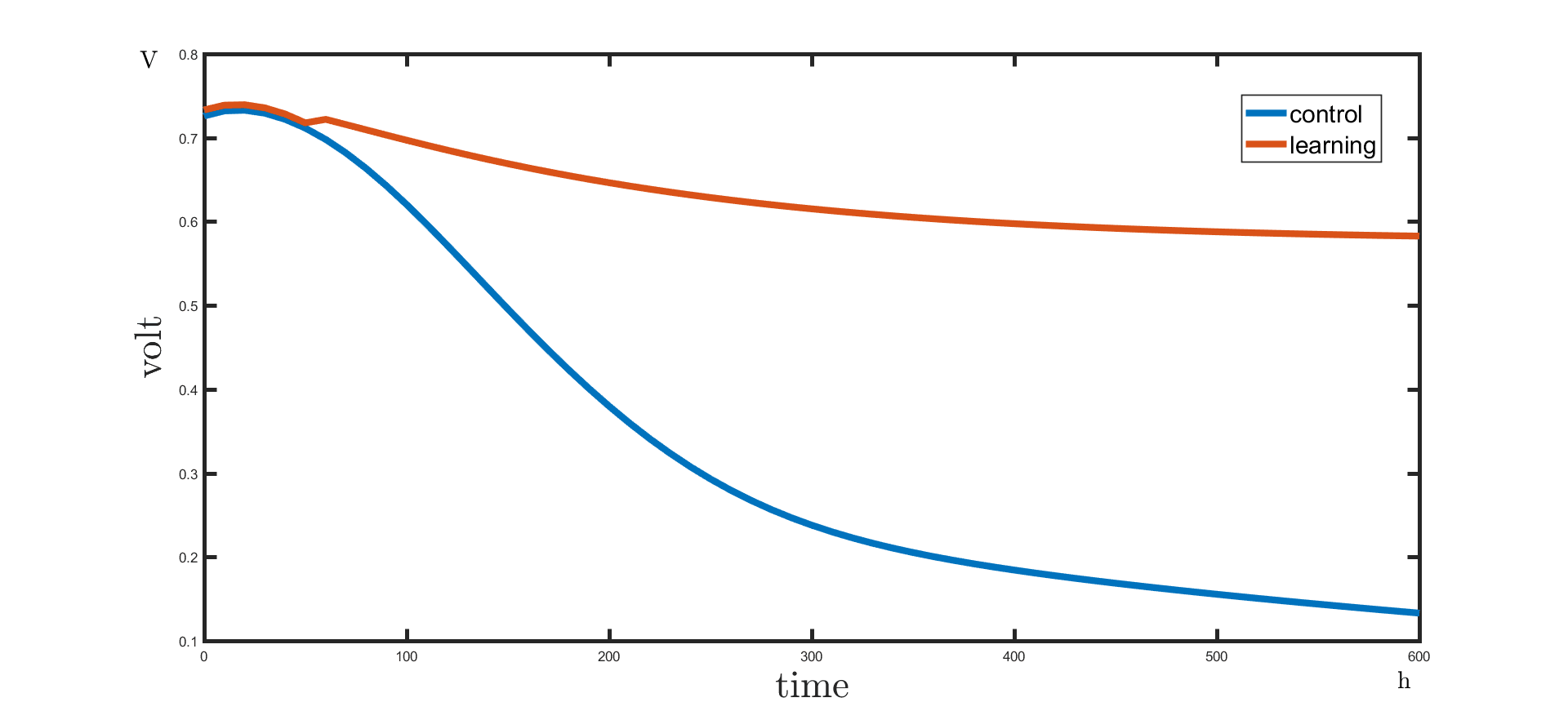# Team:HUST-China/Software

HillSide Multi purpose HTML5 Template

## Software

### Sensing module

#### Algorithms used in sensing module

Gradient descent is a iterative algorithm used find a local minimum of a function using gradient descent. If we want to fit a function h(X) (X is set of independent variable xi), loss function will be j(X). We will calculate the gradient of xi ∈ X : ∇j(X) and x(n+1)=xn-i*∇j(X).

Mean Shift

Mean shift is a non-parametric feature-space analysis technique for locating the maxima of a density function, a so-called mode-seeking algorithm (Cheng et al. 1995). This algorithm will set a core whose radius is r, each data x in core will add the move vector: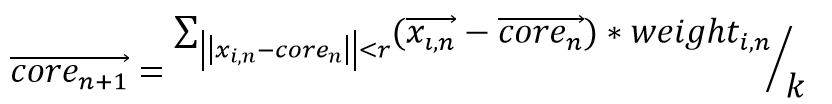Each iteration will judge and calculate a new move vector until the coordinate of the core doesn’t move.

#### Step of sensing module

Firstly, we will feed all variable’s initial value (X_0^all) and use our model to predict all variable’s value in next unit of time. Secondly, we feed the true value of variable that can be sensed (X^s). Then, we calculate the Euclidean Distance between our prediction and true value(||P_t^s [i]-X_t^s ||) and use it as weight1. Sp is the sum of predictions.

After that, we use mean shift algorithm based on the weight1 (||P_t^s [i]-X_t^s ||) to find several scores (SC). Next step is to sort possible predictions by weigh2, use gradient descent on top np predictions to get new possible prediction and kill last dp predictions. (np = new_rate *(max_prediction - sp),dp=dead_rate*sp). Finally the software start next iteration.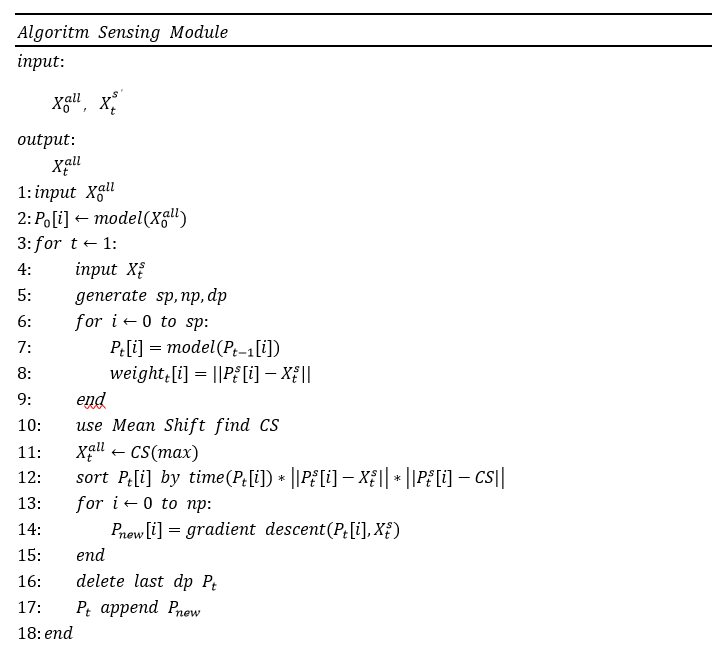#### Result

The result of sensing module is showing below, the true data is a simplified growth curve of Rhodopseudomonas, the figure shows our module works well.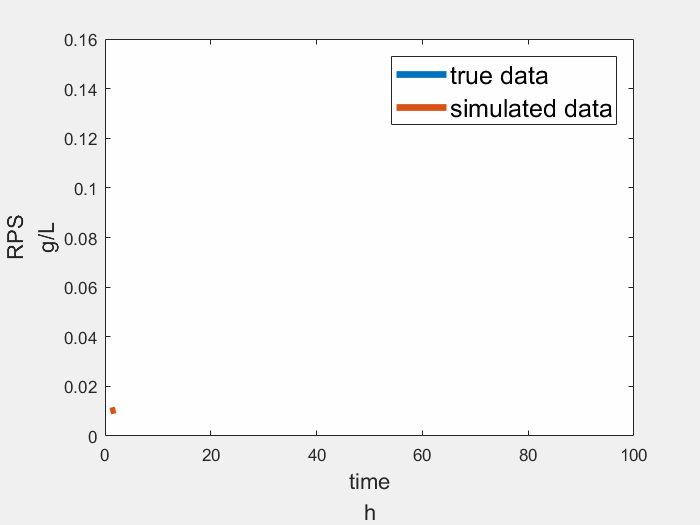### Learning Module

We use Q-Learning method to learn how to make decision based on environment. Q-learning is a reinforcement learning method used in machine learning. It can learn a policy, which tells us what actions to take under what situations. It can handle problems with stochastic transitions and rewards, without requiring adaptations.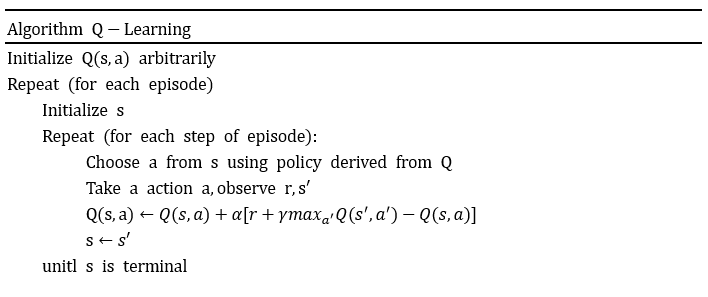#### Result

The voltage can be held in high level in a relatively long time with the control of oxygen, carbon dioxide(even lactate if needed) input by learning module. The result shows that learning module’s participation makes voltage output more stable.